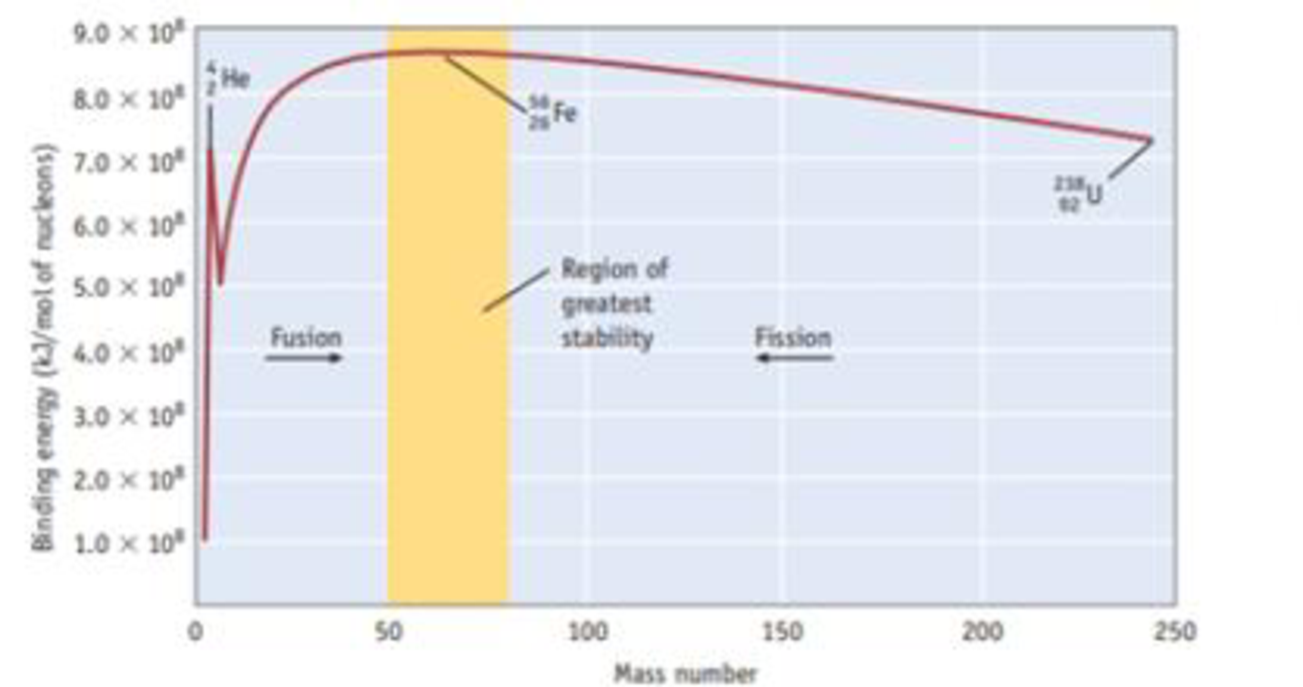# Calculate the binding energy per mole of nucleons for calcium-40, and compare your result with the value in Figure 25.4. Masses needed for this calculation are (in g/mol) H 1 1 = 1.00783, n 0 1 = 1.00867, and C 20 40 a = 39.96259. Figure 25.4 Relative stability of nuclei. Binding energy per nucleon for the most stable isotope of elements between hydrogen and uranium is plotted as a function of mass number. (Fission and fusion are discussed in Sectionc25.6.)### Chemistry & Chemical Reactivity

10th Edition
John C. Kotz + 3 others
Publisher: Cengage Learning
ISBN: 9781337399074### Chemistry & Chemical Reactivity

10th Edition
John C. Kotz + 3 others
Publisher: Cengage Learning
ISBN: 9781337399074

#### Solutions

Chapter
Section
Chapter 25, Problem 35PS
Textbook Problem

## Calculate the binding energy per mole of nucleons for calcium-40, and compare your result with the value in Figure 25.4. Masses needed for this calculation are (in g/mol) H 1 1 = 1.00783, n 0 1 = 1.00867, and C 20 40 a = 39.96259.Figure 25.4 Relative stability of nuclei. Binding energy per nucleon for the most stable isotope of elements between hydrogen and uranium is plotted as a function of mass number. (Fission and fusion are discussed in Sectionc25.6.)

Expert Solution
Interpretation Introduction

Interpretation:

Binding energy per nucleon for calcium-40 has to be calculated and the result has to be compared with the figure

Concept introduction:

Binding energy is the energy required to separate the nucleus of an atom into proton and neutron. Binding energy is given by Eb=Δm×(c)2, where Δm is the mass defect and c is the velocity of light =3×108m/sec-1

Mass defect is the sum of the masses of the individual nucleons that form an atomic nucleus and the mass of the nucleus.

Binding energy per nucleon =TotalbindingenergyofnucleusNumberofnucleonsinthenucleus

### Explanation of Solution

Given,

The mass of calcium-40=39.962589

The mass of proton=1.00783

The mass of neutrons=1.00867

Masses of the individual nucleons,

=20×1.00783=20.1566

=20×1.00867=20.1734

=20.1566+20.1734=40.33

The mass of the nucleus =39.96259

Mass defect Δm=Mm

Δm=40.33-39.96259=0.367411g/molΔm=0.367411×103kg/mol=3.67×104kg/mol

Binding energy:

Eb=Δm×(c)2=  3.67×104kg/mol×(3×108m/s)2=3.306×1013J=3.306×1010kJ

The binding energy for calcium-40 =3

### Want to see the full answer?

Check out a sample textbook solution.See solution

### Want to see this answer and more?

Bartleby provides explanations to thousands of textbook problems written by our experts, many with advanced degrees!

See solution